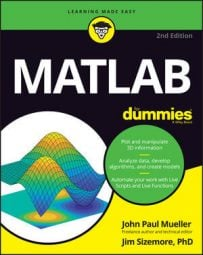##### MATLAB For DummiesYou have all sorts of ways to interact with plot data directly. By using the pause() function, in MATLAB, you can create an animation of these changes. In this case, the bars in the first row of the 3D bar chart change color one at a time. You could use such an effect during a presentation to bring focus to a particular data item.

```YSource = [1, 2, 5; 2, 4, 8; 7, 9, 10];
Bar1 = bar3(YSource);
rotate(Bar1, [0, 0, 1], 270)
Colors = get(Bar1(1), ‘CData’);
for i = 1:6:18
Colors(i,:) =[2, 2, 2, 2];
Colors(i+1,:) =[2, 2, 2, 2];
Colors(i+2,:) =[2, 2, 2, 2];
set(Bar1(1), ‘CData’, Colors);
pause(2);
Colors(i,:) =[1, 1, 1, 1];
Colors(i+1,:) =[1, 1, 1, 1];
Colors(i+2,:) =[1, 1, 1, 1];
set(Bar1(1), ‘CData’, Colors);
pause(2);
end```

The code begins by creating a 3D bar chart and rotating it so that you can see the data clearly. The code then obtains the colors used to fill the various bars from the CData property and places these values in Colors.

For this example, the CData property is an 18 × 4 matrix containing the colors for each face of the bar chart. Each bar in a chart uses six rows in the CData property.

The colors for the first bar are contained in rows 1, 2, and 3. The second bar colors appear in 7, 8, and 9, while the third bar colors appear in rows 13, 14, and 15. The other three rows in each set are hidden from view. There is a separate CData property for each of the bar groups. The example works only with the first group, Bar1(1).

Notice how this example uses the for loop. The values show that there are 18 elements. Each bar consumes six elements, so this for loop skips six elements. Instead of having the for loop set i to 1, 2, and 3, this for loop sets the values to 1, 7, and 13, which is precisely what is needed to set the color values in Colors.

After it changes the color in each of the three face elements for a particular row, the code goes on to the next row by adding 1 or 2 to the value of i. The final step is to then set the CData value in Bar1(1). You see the change occur onscreen: The bar is highlighted. After a two-second pause, the colors are returned to their original state.